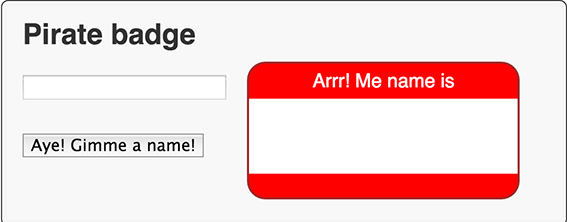# 步骤 4：创建一个 PirateName 的类

``````import 'dart:html';

import 'dart:math' show Random;

## 关键信息

• 使用 `show` 关键字，你可以只导入你需要的类，方法，和属性。
• `Random` 提供了一个随机数的发生器。

``````...
class PirateName {
}``````
• 这个类的声明给类提供一个名字

``````class PirateName {
static final Random indexGen = new Random();
}``````
• `static` 定义类级别的字段，就是说随机数发生器被所有的类实例共享。
• Dart 编辑器强调静态名字。
• 使用 `new` 调用一个构造函数。

``````class PirateName {
static final Random indexGen = new Random();
String _firstName;
String _appellation;
}``````
• 私有变量用`(_).`强调。Dart 没有 private 关键字。

``````class PirateName {
...
static final List names = [
'Anne', 'Mary', 'Jack', 'Morgan', 'Roger',
'Bill', 'Ragnar', 'Ed', 'John', 'Jane' ];
static final List appellations = [
'Jackal', 'King', 'Red', 'Stalwart', 'Axe',
'Young', 'Brave', 'Eager', 'Wily', 'Zesty'];
}``````
• `final` 修饰的变量不能更改。
• 列表是 Dart 内置的，使用 List 来创建。
• List 类提供 API 给列表。

给类提供一个构造函数。

`````` class PirateName {
...
PirateName({String firstName, String appellation}) {
if (firstName == null) {
_firstName = names[indexGen.nextInt(names.length)];
} else {
_firstName = firstName;
}
if (appellation == null) {
_appellation = appellations[indexGen.nextInt(appellations.length)];
} else {
_appellation = appellation;
}
}
}
``````
• 构造函数名和类名相同。
• 参数被包含在花括号 `({ })` 是可选的被命名的参数。
• `nextInt()` 函数得到一个随机整数从随机数发生器里。
• 使用方括号 `([ ])` 为列表添加索引。
• 使用 `length` 属性返回列表中元素的个数。
• 代码使用随机数作为列表的索引。

``````class PirateName {
...
String get pirateName =>
_firstName.isEmpty ? '' : '\$_firstName the \$_appellation';
}``````
• Getters 是一个特别的方法，提供访问对象的属性。
• 三元运算符 `?:``if-then-else` 语句的简略写法。
• 字符串插入 `('\$_firstName the \$_appellation')` 让我们很容易从其他对象构建字符串。
• 大箭头 `( => expr; )``{ return expr; }` 语法的一个简称。

``````class PirateName {
...
String toString() => pirateName;
}``````
• 因为对象实现 `toString()` 方法没有给很多的信息，很多类重写 `toString()`
• 当你调用 `print(anObject)` 得到字符串，返回值是 `anObject.toString()` 得到的。
• 重写 `toString()` 在的调试和输出的时候特别有用。

``````void setBadgeName(PirateName newName) {
}``````
• 代码调用 getter 得到 PirateName 作为一个字符串。

``````void updateBadge(Event e) {
String inputName = (e.target as InputElement).value;

...
}``````
• 调用构造函数给可选的命名参数提供一个值。

``````void generateBadge(Event e) {
}``````
• 在这种情况下，通过无参数调用构造函数。

## 运行应用